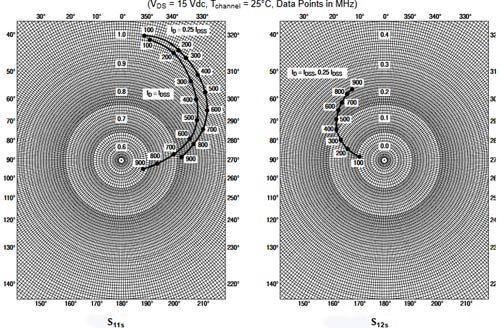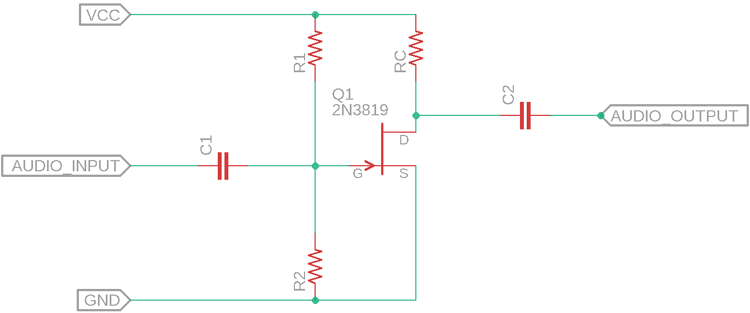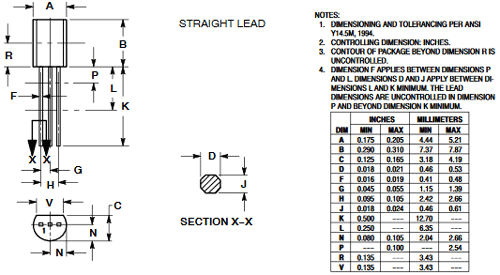# 2N3819 N-Channel JFET

[Click the image to enlarge it]

2N3819 is an N- Channel - Depletion layer JFET that is widely used in RF-based audio equipment for amplification and other purposes. It is a VHF / UHF Amplifier. It has a Drain-Source Voltage of 25 Vdc with a Drain-Gate voltage of 25 Vdc. The Gate-Source Voltage is 25V. The 2N3819 could provide 100mAdc of Drain current.

### Pin Description of 2N3819 JFET

 Pin Number Pin Name Description 1 Source Source pin of the JFET 2 Gate Gate pin of the JFET 3 Drain Drain pin of the JFET

### Features and Specification of 2N3819 JFET

• Type: JFET - N - Channel - Depletion
• Drain to Source Voltage (VDS) = 25V
• Drain to Gate Voltage (VDG) = 25V
• Gate to Source Voltage (VGS) = 25V
• Drain Current (ID)= 0.1 A
• Gate-Source Cut-off Voltage (VGS(off)) = -8.0 Vdc (VDS = 15 Vdc, ID = 10 nAdc)
• Cut-off Frequency (Note 1) = 700 Mhz (VDS = 15 Vdc, VGS = 0)

Note: Complete technical details can be found in the 2N3819 Datasheet, linked at the bottom of this page.

### Alternative Product of 2N3819 JFET

Other Replacements for 2N3819 JFET are 2N4416, NTE312, 2N5638, 2SK162, etc.

### 2N3819 JFET- Overview

The 2N3819 is an N-Channel JFET. It is suitable for VHF/UHF related RF applications where amplification is required. The below graph is the S-Parameters graph, useful to know the common source characteristic of the JFET. This JFET is also useful for switching related applications.### How to choose a JFET

1. Choose the JFET type that is required for the application. 2N3819 is an N - Channel JFET thus it is suitable to use on the low side of the power path. If the application requires high side switching, then use P-Channel.
2. Calculate the maximum Drain-Source voltage, and the Gate-Source voltage will be for the targeted application. Choose a JFET that has the Drain-Source and Gate-Source breakdown voltage more than what is actually required. 2N3819 supports 25V Drain-Source breakdown voltage and 25V Gate-Source Breakdown voltage.
3. Calculate maximum Drain Current. Choose a JFET that could tolerate more than the Drain current that is actually required for the application. 2N3819 works up to 100mA of continuous Drain current (ID).

### 2N381 - Interfacing Diagram

The below image is showing the standard application of the N-Channel JFET 2N3819 as an amplifier.The C1 is the coupling capacitor and the C2 is the DC blocking capacitor where the output will be harvested. The RC is the collector Resistor and changing this value will change the frequency response of the circuit as well as it will control the DC gain by controlling the collector current. R1 and R2 are used as a voltage divider for biasing the JFET.

A typical value for 12V operation could be: R1 = 100k, R2 = 10k, RC = 10k, and C1, C2 can be 1uF ceramic capacitors.

### Applications of 2N3819 JFET

• VHF/UHF Amplifier system
• RF Module
• Tone control
• Audio Applications

### 2D Model of 2N3819

The dimensions of 2N3819 are given below to help you with selecting the right PCB footprint for 2N3819.Component Datasheet